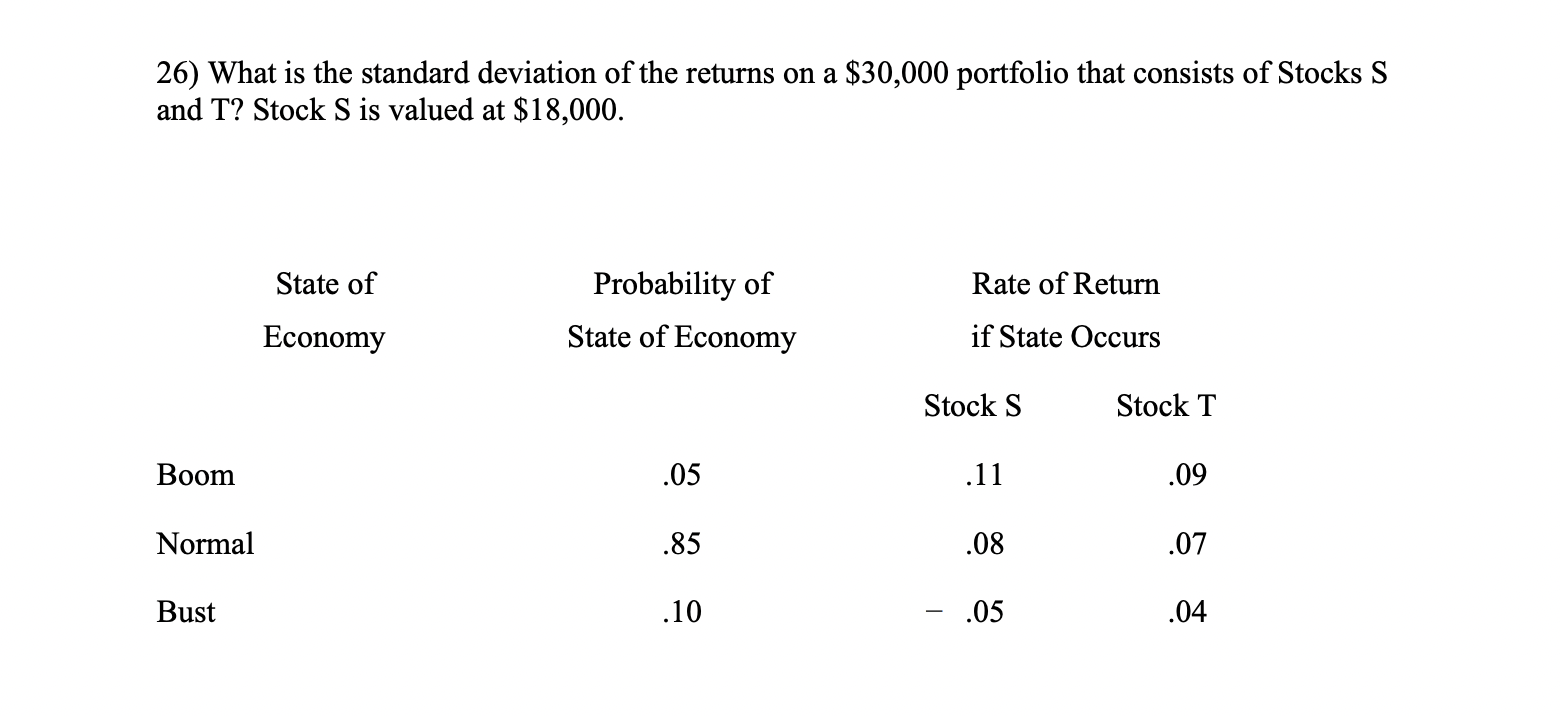# (Solved):   please solve it step by step and not on excel    26) What is the standard devi ...please solve it step by step and not on excel

26) What is the standard deviation of the returns on a $$\ 30,000$$ portfolio that consists of Stocks $$\mathrm{S}$$ and T? Stock $$\mathrm{S}$$ is valued at $$\ 18,000$$.

We have an Answer from Expert

Value of Portfolio = $30,000 Investment in Stock S =$18,000 Investment in Stock T = \$12,000 Weight of Stock S =Investment in Stock S / Value of Portf